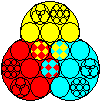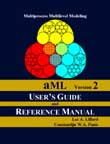Multiprocess
Multilevel ModelingaML

product info
multilevel example
multiprocess example

## aML Product Information

aML is a statistical software package for estimating multilevel, multiprocess models. Multilevel refers to the capability of handling repeated measures of outcomes; the number of levels may be arbitrarily large. Multiprocess refers to the capability of jointly estimating several outcome types, with residuals that may be correlated across outcome types. It handles a wide variety of models to support continuous outcomes, ordered and unordered categorical outcomes, duration (hazard) outcomes, and count outcomes. Many generalizations are supported, such as selection models, random coefficients models, growth models, ordered probit/logit models, sequential probit/logit models, multinomial probit/logit models, Tobit models, et cetera. All may have arbitrarily many levels and all may be mixed and matched as desired. Included with aML are a data preprocessing program and several utilities to make life easier.

aML is intuitive and easy to learn, yet very flexible. It allows you to model complex phenomena quickly, easily, and with a minimum of programming and set-up costs. Follow the links for examples of the intuitive way to specify multilevel and multiprocess models.

aML is designed for multilevel modeling. It allows for an arbitrary number of levels and for different numbers of replications at each level for each observational unit. Data may be prepared using any standard data processing package (SAS, Stata, SPSS, et cetera).

aML is designed for multiprocess modeling, i.e., joint modeling of correlated outcomes of any type. Indeed, this is its main strength and the feature that most clearly distinguishes it from other statistical estimation packages. It offers the capability to easily mix model types. Outcome types may be combined to allow joint modeling of complex processes.

aML supports the following basic outcome types and generalizations:

• Continuous outcomes: regression, Tobit (censored normal), truncated normal, seemingly unrelated regression (SUR), panel data, multiple-indicator multiple-cause (MIMC), random coefficients, multilevel, simultaneous equations;
• Ordered and unordered categorical outcomes: probit/logit, ordered probit/logit, multinomial probit/logit, sequential probit/logit, panel data, multilevel, joint/simultaneous outcomes, probits/logits with variable thresholds-in-data, probits with non-unit standard deviations;
• Duration outcome hazard models (also known as failure time models or intensity models): censored or uncensored outcomes, multilevel, time-varying variables, semiparametric baseline duration pattern, multiple duration dependencies, replication and heterogeneity, simultaneous hazards;
• Count outcomes: Poisson models, binomial models, negative binomial models, multilevel, replication with heterogeneity.
Models may be specified using "building blocks" such as regressors, residuals (normal, autoregressive and moving average, finite mixture), splines, parameters, vectors, and matrices. These building blocks may be used in multiple model equations (e.g., a probit and a hazard model equation) and interacted as desired to specify a very wide class of models. For example:
• Random coefficients model
• Structural equation model
• Truncated normal regression model
• Heckman selection model, other (multilevel) selection models
• Heteroskedasticity
• Endogenous discrete explanatory variables
• Simultaneous equations for hazards
• Endogenous switching models
aML's extensive features include multidimensional heterogeneity, Huber-corrected standard errors and covariance of estimates, weighted estimation, AR(p), MA(q) and ARMA(p,q) errors, finite mixture (Heckman-Singer) heterogeneity, and more.aML is extensively documented in a 446-page User's Guide and Reference Manual. The User's Guide discusses all basic model types and illustrates how to specify extensions and generalizations of standard models. Its numerous examples may be downloaded in electronic form from this website. The Reference Manual precisely and exhaustively documents all features.